• ## 典型相关分析CCA

热门讨论 2010-04-15 11:15:29
用于特征降维，特征融合，相关分析等多元数据分析的典型相关分析CCA）Matlab代码实现。
• 典型关联分析(Canonical Correlation Analysis，以下简称CCA)是最常用挖掘数据关联关系算法之一。比如我们拿到两组数据，第一组是人身高和体重数据，第二组是对应跑步能力和跳远能力数据。那么我们能不能...
典型关联分析(Canonical Correlation Analysis，以下简称CCA)是最常用的挖掘数据关联关系的算法之一。比如我们拿到两组数据，第一组是人身高和体重的数据，第二组是对应的跑步能力和跳远能力的数据。那么我们能不能说这两组数据是相关的呢？CCA可以帮助我们分析这个问题。

1. CCA概述

在数理统计里面，我们都知道相关系数这个概念。假设有两组一维的数据集X和Y，则相关系数ρρ的定义为:

其中是X和Y的协方差，而分别是X和Y的方差。相关系数ρ的取值为[-1,1],　ρ的绝对值越接近于1，则X和Y的线性相关性越高。越接近于0，则X和Y的线性相关性越低。

虽然相关系数可以很好的帮我们分析一维数据的相关性，但是对于高维数据就不能直接使用了。拿上面我们提到的，如果X是包括人身高和体重两个维度的数据，而Y是包括跑步能力和跳远能力两个维度的数据，就不能直接使用相关系数的方法。那我们能不能变通一下呢？CCA给了我们变通的方法。

CCA使用的方法是将多维的X和Y都用线性变换为1维的X'和Y'，然后再使用相关系数来看X'和Y'的相关性。将数据从多维变到1位，也可以理解为CCA是在进行降维，将高维数据降到1维，然后再用相关系数进行相关性的分析。下面我们看看CCA的算法思想。

2. CCA的算法思想

上面我们提到CCA是将高维的两组数据分别降维到1维，然后用相关系数分析相关性。但是有一个问题是，降维的标准是如何选择的呢？回想下主成分分析PCA，降维的原则是投影方差最大；再回想下线性判别分析LDA，降维的原则是同类的投影方差小，异类间的投影方差大。对于我们的CCA，它选择的投影标准是降维到1维后，两组数据的相关系数最大。

现在我们具体来讨论下CCA的算法思想。假设我们的数据集是X和Y，X为n1×m的样本矩阵。Y为n2×m的样本矩阵.其中m为样本个数，而n1,n2分别为X和Y的特征维度。

对于X矩阵，我们将其投影到1维，或者说进行线性表示，对应的投影向量或者说线性系数向量为aa, 对于Y矩阵，我们将其投影到1维，或者说进行线性表示，对应的投影向量或者说线性系数向量为bb, 这样X ,Y投影后得到的一维向量分别为X',Y'。我们有

我们CCA的优化目标是最大化得到对应的投影向量a,b，即

在投影前，我们一般会把原始数据进行标准化，得到均值为0而方差为1的数据X和Y。这样我们有：

由于我们的X，Y的均值均为0，则

令，则优化目标可以转化为：

由于分子分母增大相同的倍数，优化目标结果不变，我们可以采用和SVM类似的优化方法，固定分母，优化分子，具体的转化为：

也就是说，我们的CCA算法的目标最终转化为一个凸优化过程，只要我们求出了这个优化目标的最大值，就是我们前面提到的多维X和Y的相关性度量，而对应的a,ba,b则为降维时的投影向量，或者说线性系数。

这个函数优化一般有两种方法，第一种是奇异值分解SVD，第二种是特征分解，两者得到的结果一样，下面我们分别讲解。

原文地址：https://www.cnblogs.com/pinard/p/6288716.html

展开全文• ## 典型关联分析CCA

千次阅读 2017-05-17 13:15:41
在实际问题中，需要研究多个变量之间相关关系，此时，可以应用典型相关分析(Canonical Correlation Analysis)。这个算法由H.Hotelling于1936年提出，在1970年代趋于成熟。早期由于需要大量矩阵运算所以没有广泛...
前言

整理了其他人写的一些关于CCA的总结，写的真的很详细。
详见：CCA资料
刘建平_CCA总结
在实际问题中，需要研究多个变量之间的相关关系，此时，可以应用典型相关分析(Canonical Correlation Analysis)。这个算法由H.Hotelling于1936年提出，在1970年代趋于成熟。早期由于需要大量的矩阵运算所以没有广泛应用。现代计算机提高了CCA的地位。

比如，我们拿到了两组数据，第一组是人身高和体重的数据，第二组是对应的跑步能力和跳远能力的数据，那么我们可以利用CCA来分析这两组数据是否相关。

CCA概述

在数理统计中，假设有两组一维的数据集X和Y，则相关系数
ρ(X,Y)=corr（X,Y）=cov(X,Y）D(X)‾‾‾‾‾√D(Y)‾‾‾‾‾√
\rho(X,Y) =corr（X,Y）= \frac{cov(X,Y）}{\sqrt{D(X)}\sqrt{D(Y)}}

其中cov(X,Y)是X和Y的协方差，而D(X),D(Y)分别是X和Y的方差。相关系数ρ的取值为[-1,1],　ρ的绝对值越接近于1，则X和Y的线性相关性越高。越接近于0，则X和Y的线性相关性越低。

虽然相关系数可以很好的帮我们分析一维数据的相关性，但是对于高维数据就不能直接使用了。拿上面我们提到的，如果X是包括人身高和体重两个维度的数据，而Y是包括跑步能力和跳远能力两个维度的数据，就不能直接使用相关系数的方法。那我们能不能变通一下呢？CCA给了我们变通的方法。

CCA使用的方法是将多维的X和Y都用线性变换为1维的X’和Y’，然后再使用相关系数来看X’和Y’的相关性。将数据从多维变到1位，也可以理解为CCA是在进行降维，将高维数据降到1维，然后再用相关系数进行相关性的分析。下面我们看看CCA的算法思想。

CCA算法思想

我们知道，两个随机变量x、y之间的线性关系可以通过对这两个变量的N组样本对进行线性回归求得。但是，如果要求两组随机变量x、y之间的线性关系，则可以用典型关联分析（Canonical correlation analysis）来求解。CCA是寻找两组变量对应的两个线性变换wx,wy$w_x,w_y$(分别和x，y的维数相等)，使得通过线性变换后的两个组合变量（即wTxx,wTyy$w^T_x x,w^T_y y$）之间的相关系数最大。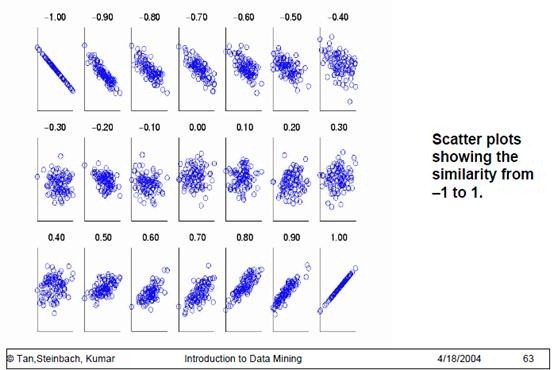现在我们具体来讨论下CCA的算法思想。假设我们的数据集是X和Y，X为m×n1$m \times n_1$的样本矩阵。Y为m×n2$m \times n_2$的样本矩阵.其中m为样本个数，而n1,n2$n_1,n_2$分别为X和Y的特征维度。

对于X矩阵，我们将其投影到1维，或者说进行线性表示，对应的投影向量或者说线性系数向量为a$a$;对于Y矩阵，我们将其投影到1维，或者说进行线性表示，对应的投影向量或者说线性系数向量为b$b$, 这样X ,Y投影后得到的一维向量分别为X’,Y’。我们有X′=aTX,Y′=bTYX' = a^TX, Y'=b^TY

我们CCA的优化目标是最大化ρ(X′,Y′)$\rho(X',Y')$得到对应的投影向量a,b$a,b$，即argmaxa,bcov(X′,Y′)D(X′)‾‾‾‾‾‾√D(Y′)‾‾‾‾‾‾√\underbrace{arg\;max}_{a,b}\frac{cov(X',Y')}{\sqrt{D(X')}\sqrt{D(Y')}}
在投影前，我们一般会把原始数据进行标准化，得到均值为0而方差为1的数据X和Y。这样我们有：
cov(X′,Y′)=cov(aTX,bTY)=E(aTX,bTY)=E((aTX)(bTY)T)=aTE(XYT)bcov(X',Y') = cov(a^TX, b^TY) = E(a^TX, b^TY) = E((a^TX)(b^TY)^T) = a^TE(XY^T)b D(X′)=D(aTX)=aTE(XXT)aD(X') = D(a^TX) = a^TE(XX^T)aD(Y′)=D(bTY)=bTE(YYT)bD(Y') =D(b^TY) = b^TE(YY^T)b

由于我们的X，Y的均值均为0，则D(X)=cov(X,X)=E(XXT),D(Y)=cov(Y,Y)=E(YYT)D(X) = cov(X,X) = E(XX^T), D(Y)= cov(Y,Y) = E(YY^T)cov(X,Y)=E(XYT),cov(Y,X)=E(YXT)cov(X,Y) = E(XY^T), cov(Y,X) = E(YX^T)

令SXY=cov(X,Y)$S_{XY} =cov(X,Y)$，则优化目标可以转化为：argmaxa,baTSXYbaTSXXa‾‾‾‾‾‾‾√bTSYYb‾‾‾‾‾‾‾√\underbrace{arg\;max}_{a,b}\frac{a^TS_{XY}b}{\sqrt{a^TS_{XX}a}\sqrt{b^TS_{YY}b}}

由于分子分母增大相同的倍数，优化目标结果不变，我们可以采用和SVM类似的优化方法，固定分母，优化分子，具体的转化为：argmaxa,baTSXYb\underbrace{arg\;max}_{a,b}\;\;{a^TS_{XY}b}s.t.aTSXXa=1,bTSYYb=1s.t. a^TS_{XX}a =1,\; b^TS_{YY}b =1

也就是说，我们的CCA算法的目标最终转化为一个凸优化过程，只要我们求出了这个优化目标的最大值，就是我们前面提到的多维X和Y的相关性度量，而对应的a,b$a,b$则为降维时的投影向量，或者说线性系数。

这个函数优化一般有两种方法，第一种是奇异值分解SVD，第二种是特征分解，两者得到的结果一样。

CCA算法的SVD求解

对于上面的优化目标，我们可以做一次矩阵标准化，就可以用SVD来求解了。
首先，我们令a=S−1/2XXu,b=S−1/2YYv$a=S_{XX}^{-1/2}u, b=S_{YY}^{-1/2}v$,这样我们有：aTSXXa=1⇒uTS−1/2XXSXXS−1/2XXu=1⇒uTu=1a^TS_{XX}a =1 \Rightarrow u^TS_{XX}^{-1/2}S_{XX}S_{XX}^{-1/2}u =1\Rightarrow u^Tu=1bTSYYb=1⇒vTS−1/2YYSYYS−1/2YYv=1⇒vTv=1b^TS_{YY}b =1 \Rightarrow v^TS_{YY}^{-1/2}S_{YY}S_{YY}^{-1/2}v=1 \Rightarrow v^Tv=1aTSXYb=uTS−1/2XXSXYS−1/2YYva^TS_{XY}b = u^TS_{XX}^{-1/2}S_{XY}S_{YY}^{-1/2}v
也就是说，我们的优化目标变成下式：argmaxu,vuTS−1/2XXSXYS−1/2YYv\underbrace{arg\;max}_{u,v}u^TS_{XX}^{-1/2}S_{XY}S_{YY}^{-1/2}vs.t.uTu=1,vTv=1s.t. u^Tu =1,\; v^Tv =1

仔细一看，如果将u和v看做矩阵M=S−1/2XXSXYS−1/2YY$M=S_{XX}^{-1/2}S_{XY}S_{YY}^{-1/2}$的某一个奇异值对应的左右奇异向量。那么利用奇异值分解，我们可以得到M=UΣVT$M=U\Sigma V^T$,其中U,V$U,V$分别为M的左奇异向量和右奇异向量组成的矩阵，而Σ$\Sigma$为M的奇异值组成的对角矩阵。由于U,V$U,V$所有的列都为标准正交基，则uTU$u^TU$和VTv$V^Tv$得到一个只有一个标量值为1，其余标量值为0的向量。此时我们有uTS−1/2XXSXYS−1/2YYv=uTUΣVTv=σuvu^TS_{XX}^{-1/2}S_{XY}S_{YY}^{-1/2}v = u^TU\Sigma V^Tv = \sigma_{uv}

也就是说我们最大化uTS−1/2XXSXYS−1/2YYv$u^TS_{XX}^{-1/2}S_{XY}S_{YY}^{-1/2}v$,其实对应的最大值就是某一组左右奇异向量所对应的奇异值的最大值。也就是将M做了奇异值分解后，最大的奇异值就是我们优化目标的最大值，或者说我们的X和Y之间的最大相关系数。利用对应的左右奇异向量u,v$u,v$我们也可以求出我们原始的X和Y的线性系数a=S−1/2XXu,b=S−1/2YYv$a=S_{XX}^{-1/2}u, b=S_{YY}^{-1/2}v$。

可以看出，SVD的求解方式非常简洁方便。但是如果不熟悉SVD的话，也可以用传统的拉格朗日函数加上特征分解来完成这个函数的优化。

CCA算法的特征分解求解

特征分解方式就比较传统了，利用拉格朗日函数，优化目标转化为最大化下式：J(a,b)=aTSXYb−λ2(aTSXXa−1)−θ2(bTSYYb−1)J(a,b) = a^TS_{XY}b -\frac{\lambda}{2}(a^TS_{XX}a-1)-\frac{\theta}{2}(b^TS_{YY}b-1)

分别对a,b$a,b$求导并令结果为0，我们得到：SXYb−λSXXa=0S_{XY}b-\lambda S_{XX}a=0SYXa−λSYYb=0S_{YX}a-\lambda S_{YY}b=0
将上面第一个式子左乘aT$a^T$,第二个式子左乘bT$b^T$，并利用aTSXXa=1,bTSYYb=1$a^TS_{XX}a =1,\; b^TS_{YY}b =1$，我们得到λ=θ=aTSXYb\lambda = \theta = a^TS_{XY}b
其实也就是说我们的拉格朗日系数就是我们要优化的目标。我们继续将上面的两个式子做整理，第一个式子左乘S−1XX$S_{XX}^{-1}$,第二个式子左乘S−1YY$S_{YY}^{-1}$，我们得到：S−1XXSXYb=λaS_{XX}^{-1}S_{XY}b=\lambda aS−1YYSYXa=λbS_{YY}^{-1}S_{YX}a =\lambda b
将上面第二个式子带入第一个式子，我们得到S−1XXSXYS−1YYSYXa=λ2aS_{XX}^{-1}S_{XY}S_{YY}^{-1}S_{YX}a=\lambda^2a
这个式子我们就熟悉了，这不就是特征分解吗！要求最大的相关系数λ$\lambda$,我们只需要对矩阵N=S−1XXSXYS−1YYSYX$N=S_{XX}^{-1}S_{XY}S_{YY}^{-1}S_{YX}$做特征分解，找出最大的特征值取平方根即可，此时最大特征值对应的特征向量即为X的线性系数a$a$。

同样的办法，我们将上面第一个式子带入第二个式子，我们得到S−1YYSYXS−1XXSXYb=λ2bS_{YY}^{-1}S_{YX}S_{XX}^{-1}S_{XY}b=\lambda^2b,只需要对矩阵N′=S−1YYSYXS−1XXSXY$N’=S_{YY}^{-1}S_{YX}S_{XX}^{-1}S_{XY}$做特征分解，找出最大的特征值取平方根即可，此时最大特征值对应的特征向量即为Y的线性系数b$b$。

可以看出特征分解的方法要比SVD复杂，但是两者求得的结果其实是等价的，只要利用SVD和特征分解之间的关系就很容易发现两者最后的结果相同。

CCA算法流程

这里我们对CCA的算法流程做一个总结，以SVD方法为准。

输入：各为m个的样本X和Y，X和Y的维度都大于1 输出：X,Y的相关系数ρ$\rho$,X和Y的线性系数向量a和b
1）计算X的方差SXX$S_{XX}$, Y的方差SYY$S_{YY}$，X和Y的协方差SXY$S_{XY}$,Y和X的协方差SYX=STXY$S_{YX}=S_{XY}^T$
2) 计算矩阵M=S−1/2XXSXYS−1/2YY$M=S_{XX}^{-1/2}S_{XY}S_{YY}^{-1/2}$
3）对矩阵M$M$进行奇异值分解，得到最大的奇异值ρ$\rho$，和最大奇异值对应的左右奇异向量u,v$u,v$
4) 计算X和Y的线性系数向量a和b, a=S−1/2XXu,b=S−1/2YYv$a=S_{XX}^{-1/2}u, b=S_{YY}^{-1/2}v$

小结

CCA算法广泛的应用于数据相关度的分析，同时还是偏最小二乘法的基础。但是由于它依赖于数据的线性表示，当我们的数据无法线性表示时，CCA就无法使用，此时我们可以利用核函数的思想，将数据映射到高维后，再利用CCA的思想降维到1维，求对应的相关系数和线性关系，这个算法一般称为KCCA。
此外，我们在算法里只找了相关度最大的奇异值或者特征值，作为数据的相关系数，实际上我们也可以像PCA一样找出第二大奇异值，第三大奇异值，。。。得到第二相关系数和第三相关系数。然后对数据做进一步的相关性分析。但是一般的应用来说，找出第一相关系数就可以了。
有时候我们的矩阵SXX,SYY$S_{XX},S_{YY}$不可逆，此时我们得不到对应的逆矩阵，一般遇到这种情况可以对SXX,SYY$S_{XX},S_{YY}$进行正则化，将SXX,SYY$S_{XX},S_{YY}$变化为SXX+γI,SYY+γI$S_{XX}+\gamma I,S_{YY}+\gamma I$,然后继续求逆。其中γ$\gamma$为正则化系数。展开全文算法
• 最近有小伙伴在问我一个数据分析的问题, 做毕设, 实证分析. 不知道改如何处理数据.看了下设计量表大致是这样, 都是 5级里克特量表, 大致分为两波, X, Y. 小伙伴认为就只有两个变量, 这是从商业理论上来认识,...
最近有小伙伴在问我一个数据分析的问题, 做毕设, 实证分析. 不知道改如何处理数据.看了下设计的量表大致是这样的, 都是 5级的里克特量表, 大致分为两波, X, Y. 小伙伴认为就只有两个变量, 这是从商业理论上来认识的, 但从数据的角度, 却不是的.X: 一共有22个问题, 也就是22个字段;  里面又是有认为分组的, 三两个字段, 又被认定为一个别名.Y: 一共有13个问题, 也就是13个字段; 里面有是人为分组, 三两字段啥的, 分为 4组, 分别有别名.然后不知道该如何分析?问题探寻 X 与 Y 的相关关系(线性相关)其实探讨的时候, 挺不易的, 就很难知道她到底想要分析什么, 需求是什么, 还以为要做什么回归分析, 什么相关分析, 什么统计描述或其他的, 总之, 沟通过程非常漫长. 最后我放弃了, 还是单纯从这个数据级来分析.本质上, 其实宏观来看, 就是 X 和 Y 的相关性如何嘛, 以及如何影响的. 那这不是求一波, 相关系数嘛. 但这里, X, Y 是多个字段, 是多对多 的关系, 就求不来了. 因此需要引进新的方法.CCA于是引入了典型相关分析 (Canonical Correlation Analysis), 用于探索多变量之间的关联关系.于是这个问题, 就可以初步这样来做.更正一波,写的有点不对, 不是分别降低到一维度. 而是分别降维后, x 和 y 能进行 配对. 这里 y 有13个嘛, x 有22个, 假设根本不对 y 进行降维, 那最多也只能匹配到 13对. 约束条件就是相关系数最大呀.  这块的数学公式就暂时不写了, 跟 PCA , 因子分析的逻辑是类似的.发现了一个神器, 在线SPSS, 叫做 SPSSAU, 付费的, 但功能强大, UI 很有感觉, 重点是完全实现 傻瓜式操作. 虽然我已经不再做这块了, 但还是很怀念 SPSS, 比较是我数据分析之路的启蒙软件. 至少是真正用来做数据分析, 做市场研究的.简单, 托拉拽, 一键输出报告, 包含 假设检验. 探寻数据的应用意义, 而不用太多关注底层的数学公式. 虽然数学公式会更加帮助理解数据集, 这是后话. 我觉得这才是数据分析的意义:描述性统计分析关联性统计分析探索性建模分析这种基于统计理论的分析框架 + 商业理论, 已早已熟练于心. 虽然现在的不用这类 傻瓜工具了, 现在自己搞编程, 但我感觉企业中的数据分析, 至少我接触的反而更加低级.写 sql 查询数据 或 手动下载数据筛选字段, 合并表格计算业务指标, 几遍的加减乘除, 什么同比环比大量的分组聚合, 生成报表, 看板真的是, 从技术层面, 毫无难度. 我很多时间都是干这些活, 相比数据分析,我认为的, 我感觉还真不如几年前用 SPSS 的时光. 起码是真的再利用数据的价值来进行市场研究, 市场分析.然后会最终得到这样类似的结果 , 和一些假设检验, 因子载荷等的术语, 都蛮简单的. (我没跑, 数据暂不能公开, 找了一张网上的示意图)这样 CCP 就完成了, 多自变量 和 多因变量的关联分析了.Next - 回归继续要探寻, X 和部分 y  的关系.  我的思路, 都既然做相关分析了, 那很自然再拓展到回归分析呀.合并 y 为 1 列回归分析的 y 是一个字段, 因此, 可以将 量表中的 小 y 组进行, 合并为一列. 这里, 可以加权 或者 直接平均, 自己能解释清楚就行.主成分 + 多元回归有一个 y,  有很多的 x1, x2, x2...  相关分析, 就是要判断, 这些 x1, x2..与 y 是都是分别有线性相关性的(相关系数高);  而  x1, 与 x2, x3.. 之间呢, 彼此相关系数 要低第二步就是要降维. 为啥必须要降维度呢, 就是怕 X 矩阵, 存在共线, 然后就不能 求 逆了呀.PCA降维至于如何降维, 我感觉我自己都说烂了. 也搞好几年了, 就是让特征重新进行线性组合 (改变数据了哦) 为几个较少得到特征, 然后尽可能保留原来更多的信息 (协方差的范数尽可能大)求解模型参数方法1 是一步求解, 就用上面的共线图中的矩阵运算即可.方法2 是用梯度下降法来做, 我用的多, 但这个小伙伴, 没有学过编程, 就还是给推荐, 撒花是点点点算了.小结多自变量 和 多因变量 分析可以考虑 典型相关分析 CCA 这种 "降维配对" 的技术回归分析必须 3步:  先做相关性分析; 再做降维处理; 再训练模型参数;PCA 我感觉非常厉害的. 还有一在线版spssau 的工具体验感很好, 市场研究方面的数据处理, 很适合.
展开全文• 文章来源于"脑机接口社区"CCA典型关联分析原理与Python案例​mp...CCA典型相关分析CCA(canonical correlation analysis)利用综合变量对之间相关关系来反映两组指标之间整体相关性多元统计分析方法。它基本原...
文章来源于"脑机接口社区"CCA典型关联分析原理与Python案例​mp.weixin.qq.com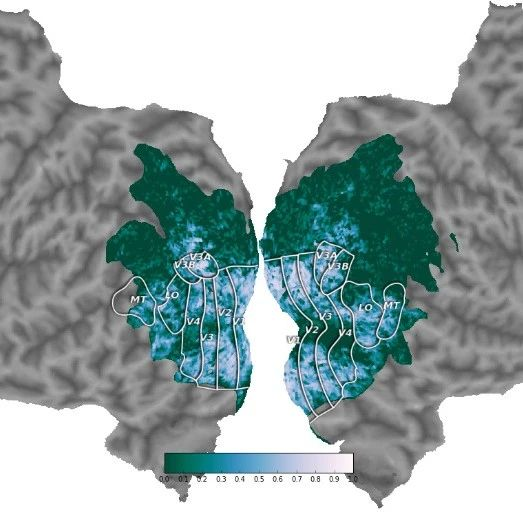Rose今天分享一下CCA的相关原理以及Python应用，CCA在EEG等脑电数据的特征提取中使用很多，很有必要熟悉其原理。CCA典型相关分析CCA(canonical correlation analysis)利用综合变量对之间的相关关系来反映两组指标之间的整体相关性的多元统计分析方法。它的基本原理是：为了从总体上把握两组指标之间的相关关系，分别在两组变量中提取有代表性的两个综合变量U1和V1（分别为两个变量组中各变量的线性组合），利用这两个综合变量之间的相关关系来反映两组指标之间的整体相关性。1936年，Hotelling提出典型相关分析。考虑两组变量的线性组合, 并研究它们之间的相关系数p(u,v).在所有的线性组合中, 找一对相关系数最大的线性组合, 用这个组合的单相关系数来表示两组变量的相关性, 叫做两组变量的典型相关系数, 而这两个线性组合叫做一对典型变量。在两组多变量的情形下, 需要用若干对典型变量才能完全反映出它们之间的相关性。下一步, 再在两组变量的与u1,v1不相关的线性组合中, 找一对相关系数最大的线性组合, 它就是第二对典型变量, 而且p(u2,v2)就是第二个典型相关系数。这样下去, 可以得到若干对典型变量, 从而提取出两组变量间的全部信息。典型相关分析的实质就是在两组随机变量中选取若干个有代表性的综合指标（变量的线性组合）, 用这些指标的相关关系来表示原来的两组变量的相关关系。这在两组变量的相关性分析中, 可以起到合理的简化变量的作用; 当典型相关系数足够大时, 可以像回归分析那样, 由- 组变量的数值预测另一组变量的线性组合的数值。原理描述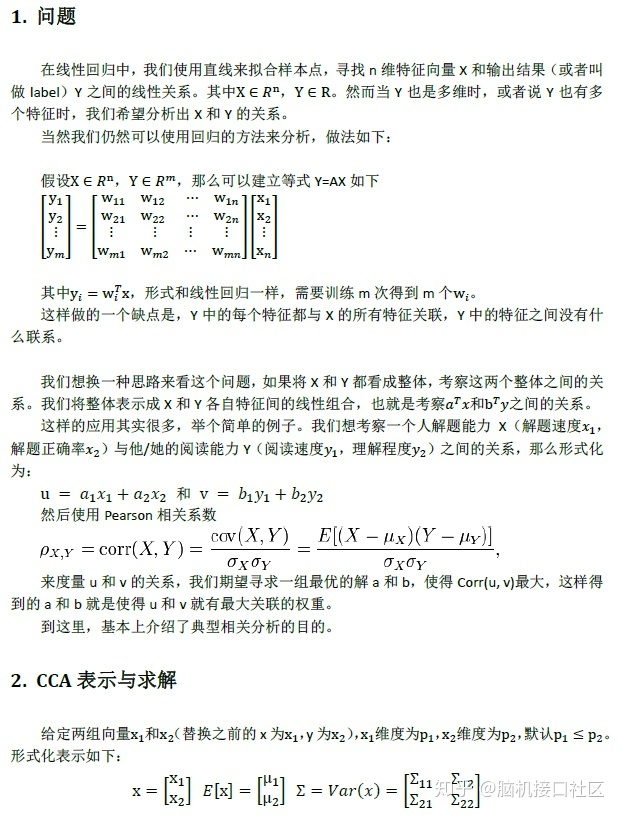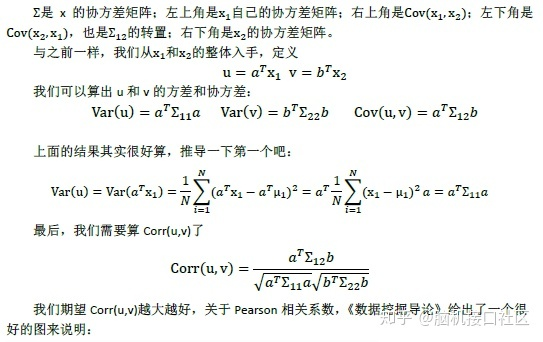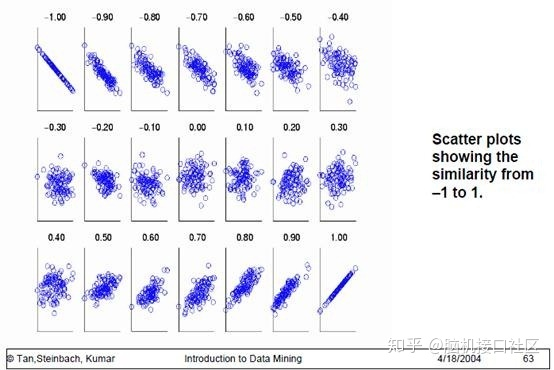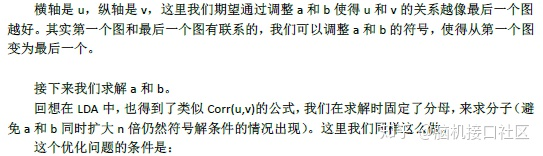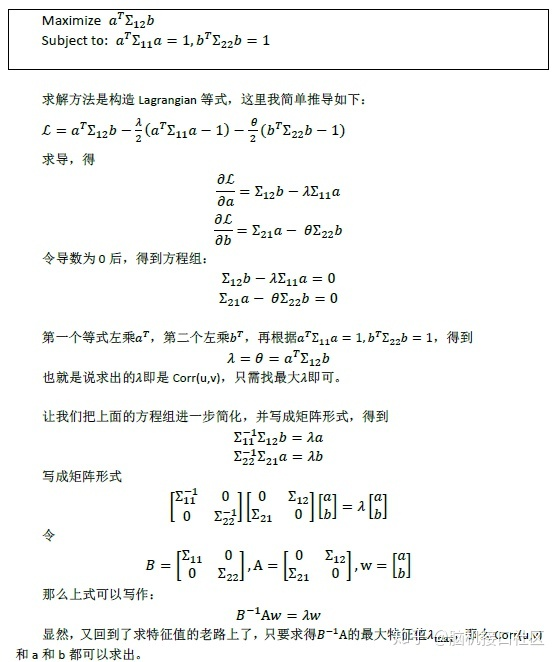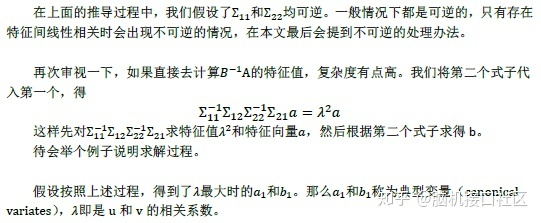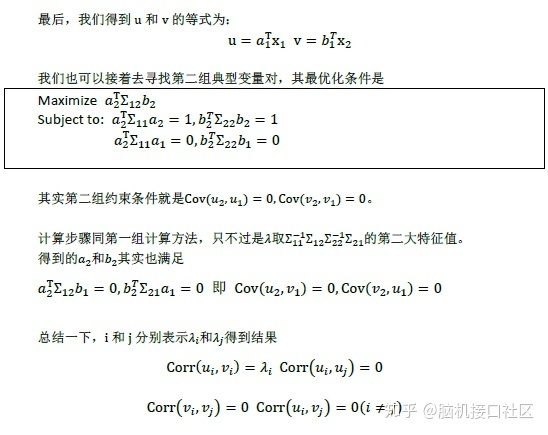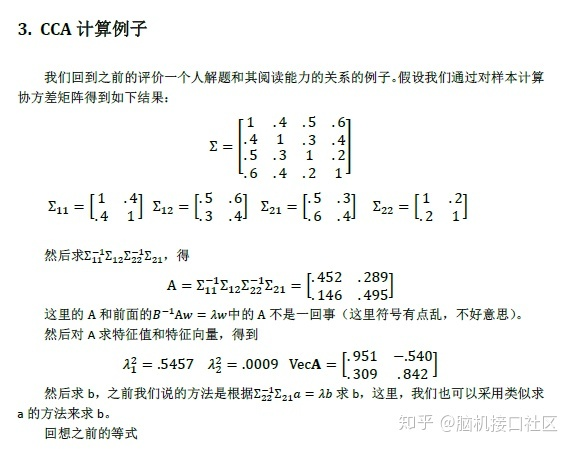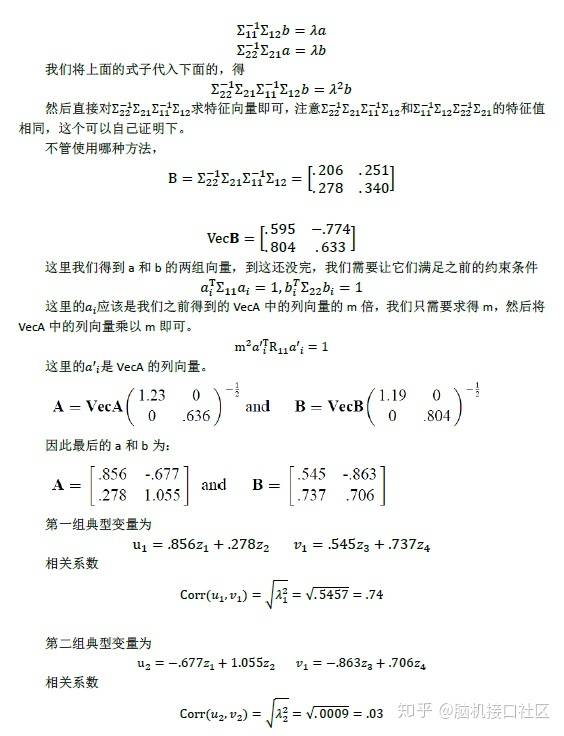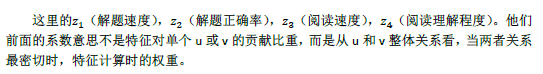案例实现# 导入工具包
import h5py
import rcca
import sys
import numpy as np
import cortex
zscore = lambda d: (d-d.mean(0))/d.std(0)第一步：加载数据请从CRCNS下载数据：http://crcns.org/data-sets/vc/vim-2以下分析假定该数据位于当前目录中名为“ data”的目录中。data = []
vdata = []
numSubjects = 3
# subjects 是3个受试者列表.
subjects = ['S1', 'S2', 'S3']
# xfms 是Pycortex中变换名称的列表，该名称用于对齐每个受试者的功能和解剖数据。
xfms = ['S1_xfm', 'S2_xfm', 'S3_xfm']
dataPath ="./data/VoxelResponses_subject%d.mat"
for subj in range(numSubjects):
# 打开数据
f = h5py.File(dataPath % (subj+1),'r')
#  获取数据大小
datasize = (int(f["ei"]["datasize"].value),int(f["ei"]["datasize"].value),int(f["ei"]["datasize"].value))
# 从Pycortex获取皮质面罩
# 获取该受试者的训练数据
data_subj = np.nan_to_num(zscore(np.nan_to_num(f["rt"].value.T)))
# 获取受试者的验证数据
vdata_subj = np.nan_to_num(zscore(np.nan_to_num(f["rv"].value.T)))
vdata.append(vdata_subj.reshape((vdata_subj.shape,)+datasize)[:, mask])第二步：定义CCA参数# 这里设置1e-4和1e2之间的一系列正则化值
regs = np.array(np.logspace(-4, 2, 10))

# 这里考虑3到10之间的成分数量
numCCs = np.arange(3, 11)

# 初始化cca模型
cca = rcca.CCACrossValidate(numCCs=numCCs, regs=regs)第三步：对数据训练，分析并保存分析结果"""
说明：
由于数据量大，此分析的计算量很大。
在笔记本中运行它会花费大量时间，因此建议对其进行并行化和/或在计算机群集上运行它，然后加载结果以进行可视化。
"""

# 利用cca训练数据
cca.train(data)

# 利用cca对验证数据进行验证
cca.validate(vdata)

# 计算方差，解释每个体素中的验证响应
cca.compute_ev(vdata)

# 保存分析结果
cca.save("./data/CCA_results.hdf5")第四步：可视化分析结果# 导入可视化工具包
%matplotlib inline
import matplotlib.pyplot as plt

# 导入Brewer色彩图以进行可视化
from brewer2mpl import qualitative

nSubj = len(cca.corrs)
nBins = 30
bmap = qualitative.Set1[nSubj]
f = plt.figure(figsize = (8, 6))
for s in range(nSubj):
# 绘制所有三个对象的所有体素之间的相关性直方图
ax.hist(cca.corrs[s], bins = nBins, color = bmap.mpl_colors[s], histtype="stepfilled", alpha = 0.6)
plt.legend(['Subject 1', 'Subject 2', 'Subject 3'], fontsize = 16)
ax.set_xlabel('Prediction correlation', fontsize = 20)
ax.set_ylabel('Number of voxels', fontsize = 20)
ax.set_title("Prediction performance across voxels", fontsize = 20)
# p <0.05时的显着性阈值(针对多次比较进行了校正)
# 重要性是使用渐近方法计算的（有关详细信息，请参见论文文本）
thresh = 0.0893
ax.axvline(x = thresh, ymin = 0, ymax = 7000, linewidth = 2, color = 'k')
ax.text(thresh+0.05, 5000, 'p<0.05', fontsize = 16)
ax.set_xticklabels(0.1*np.arange(-8, 11, 2), fontsize = 16)
ax.set_yticklabels(np.arange(0, 10000, 1000), fontsize = 16)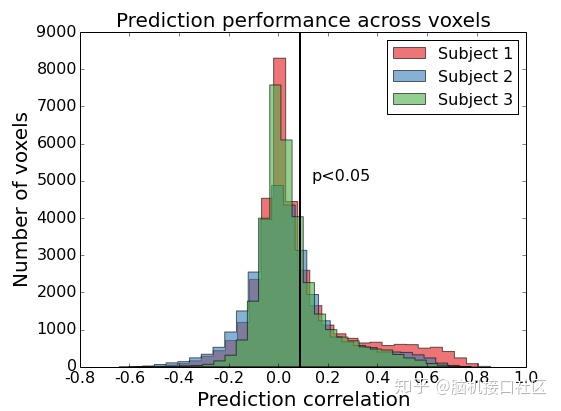该图显示了皮质图上一个对象的跨学科预测结果，即预测的验证响应和实际的验证响应之间的相关性。不重要的相关性（p <0.05，已针对多个比较进行校正）设置为0。import cortex
from matplotlib import cm
from copy import deepcopy
subj = 0
subjName = "S1"
subjTransform = "S1_xfm"
corrs = deepcopy(cca.corrs[subj])
# 将所有低于显着性阈值的体素设置为0
corrs[corrs<thresh] = 0
_ = cortex.quickflat.make_figure(cortex.Volume(corrs, subjName,
subjTransform,
cmap = cm.PuBuGn_r,
vmin = 0., vmax = 1.), with_curvature = True)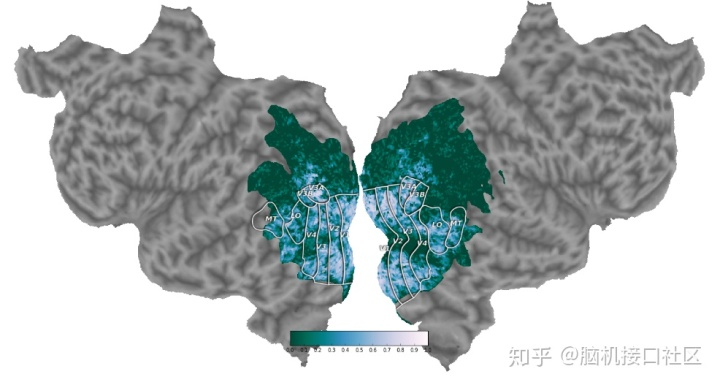参考脑机接口社区：CCA典型关联分析原理与Python案例点击上面动图扫描二维码，关注我们
展开全文• ## 典型关联分析(CCA)

千次阅读 2018-12-03 19:56:46
文章目录典型关联分析(CCA)CCA概述CCA的算法思想CCA算法SVD求解CCA算法特征分解求解CCA算法流程CCA算法小结 典型关联分析(CCA) 转载来自 刘建平Pinard https://www.cnblogs.com/pinard/p/6288716.html 典型...
• 对应分析典型相关分析笔记_数学建模系列这里对应分析典型相关分析仍然用于降维，因子分析的进阶！ 对应分析：在同一张图上，直观展现样本和属性聚类效果，同时省去因子选择、因子轴旋转等复杂过程。具体...数学建模 R
• 相关分析是研究两两变量间关系方法，在现实生活中，...典型相关分析CCA）用于研究一组X与一组Y数据之间相关关系情况。它是借助主成分分析思想，从两组变量中提取出一个或少数几个综合变量（即典型变量），从...变化检测 机器学习 MAD
• 文章来源于"脑机接口社区"CCA典型关联分析原理与Python案例​mp...CCA典型相关分析CCA(canonical correlation analysis)利用综合变量对之间相关关系来反映两组指标之间整体相关性多元统计分析方法。它基本原...
• CCA———典型相关分析，很经典论文，需要拿走，但是因为我也是菜鸟，不得已，还是得要点分。
• 先看两个数学概念： ...相关关系是一种非确定性关系，相关系数是研究变量之间线性相关程度量。由于研究对象不同，相关系数有如下几种定义方式： 简单相关系数：又叫相关系数或线性相关系数，一般用字母机器学习
• 典型相关分析（Canonical Correlation Analysis）是研究两组变量之间相关关系一种多元统计方法，它能够揭示出两组变量之间内在联系。         在一元统计分析中，用相关系数来...
• 1.典型相关(CCA)基本原理1.1典型相关概念典型相关分析是研究两组变量之间关系一种多变量统计分析方法，它可以反映两组变量之间相互依赖线性关系。设两组变量用x1，x2…xp，及y1，y2….yq表示，采用类似...
• ## CCA典型关联分析

千次阅读 2017-09-05 18:36:54
典型关联分析（Canonical Correlation Analysis） [pdf版本] 典型相关分析.pdf 1. 问题  在线性回归中，我们使用直线来拟合样本点，寻找n维特征向量X和输出结果（或者叫做label）Y之间线性关系。其中，。...
• 典型关联分析CCA）算法原理、问题提出、算法实现
• 典型关联分析(Canonical Correlation Analysis，以下简称CCA)是最常用挖掘数据关联关系算法之一。比如我们拿到两组数据，第一组是人身高和体重数据，第二组是对应跑步能力和跳远能力数据。那么我们能不能...多视角学习 机器学习 数据融合
• ## 典型相关分析原理（CCA）

千次阅读 多人点赞 2020-01-21 12:29:17
CCA典型相关分析 （canonical correlation analysis）利用综合变量对之间相关关系来反映两组指标之间整体相关性多元统计分析方法。它基本原理是：为了从总体上把握两组指标之间相关关系，分别在两组变量中...
• 典型相关性分析英文讲义，包括应用背景讲解，理论基础，典型相关项分析算法详解及典型相关性分析的拓展算法讲解
• ## CCA:典型相关分析

千次阅读 2019-07-02 14:09:06
首先，什么是非典型的即经典的相关分析。给你两组变量X=[x1,x2,...,xm]，Y=[y1,y2,...,yn]，要研究X和Y之间的相关性，就是要得到一个X和Y的协方差矩阵cov(X,Y)，矩阵中的每一个值uij表示的就是X的第i维特征xi和Y的...
• 典型相关分析 摘要 利用典型相关分析的思想 , 提出了解决了当两组特征矢量构成 总体协方差矩阵奇异时 , 典型投影矢量集求解问题 , 使之适合于高 维小样本情形 , 推广了典型相关分析的适用范围 . 首先 , 探讨了...
• CCA典型相关分析 （canonical correlation analysis）利用综合变量对之间相关关系来反映两组指标之间整体相关性多元统计分析方法。它基本原理是：为了从总体上把握两组指标之间相关关系，分别在两组变量......